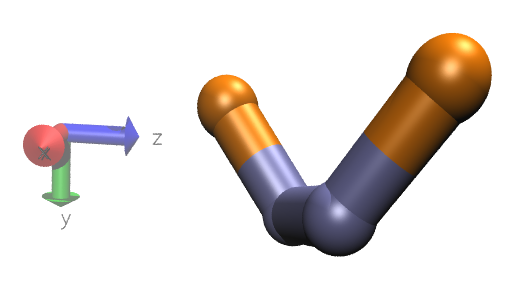# Possible error in dihedral_class2.cpp Lammps source code

Dear Everyone,

After checking the dihedral_class2.cpp in the latest version Lammps, I found a conflict between the standard convention for defining the sign of the dihedral angle and the one implemented in dihedral_class2.

The standard convection: http://lammps.sandia.gov/doc/Eqs/dihedral_sign.jpg

http://kinemage.biochem.duke.edu/teaching/anatax/pdfs/AnaTax_I.B.pdf

But in dihedral_class2, it was implemented the opposite:

// addition by Andrew Jewett, Jan 2013

// adjust the sign of phi if necessary for negative input angles

// n123 = vb2 x vb1

double n123x = vb1yvb2z - vb1zvb2y;

double n123y = vb1zvb2x - vb1xvb2z;

double n123z = vb1xvb2y - vb1yvb2x;

double n123_dot_vb3 = n123xvb3x + n123yvb3y + n123z*vb3z;

if (n123_dot_vb3 > 0.0) {

phi = -phi;

sinphi = -sinphi;

}

which says the positive phi points into page. Is it an error?

Thank you for your time and comment,

Lili

Hello All,

I hope the email below finds you well. Can anyone please help clear up my doubt? Thank you very much. Lili

Dear Lili and John

It is always confusing to tell the dihedral-angle sign from the
formula in the code. However, all of the dihedral styles (as of
2013-1) obey this sign convention:
http://lammps.sandia.gov/doc/dihedral_style.html

This seems to be equivalent to the link from your earlier post:
http://kinemage.biochem.duke.edu/teaching/anatax/pdfs/AnaTax_I.B.pdf

In any case I just tested "dihedral_style class2" again using the
Jan20 code, and the sign looks consistent. Please check the attached
image. (The angle in the image should be -60 degrees. Does this look
okay to you?)

---- testing the dihedral angle ----

You can test the dihedral angle sign convention yourself by
(Note: Please ignore the text from that post. That issue was

lmp_linux -i in.test
(assuming "lmp_linux" is your lammps binary)

This is a simple thermal annealing simulation of 4 consecutively
bonded atoms. During the simulation, the shape should relax to energy
minimum located at phi1=-60 degrees. The dihedral forces were set
with this command ("in.test"):

dihedral_coeff 1 10.0 -60 0.0 -60 0.0 -60
(http://lammps.sandia.gov/doc/dihedral_class2.html)

Results:
See attached file "test_class2_phi1=-60degrees.png".

I am using the Jan20 2014 version of LAMMPS, which uses the same code
you posted. This seems to be consistent with the sign convention that
you posted. (Again, the angle shown in the picture is -60 degrees,
not 60. You must use viewing software which uses a right-handed
coordinate system http://i.msdn.microsoft.com/dynimg/IC412518.png
I am using VMD.)Dear Andrew,

Thanks for your detail explanation. I calculated the dihedral angle by using the 4 atoms’ coordinates in butane.data file from your folder “test_dihedral_class2.tar.gz.”
Atoms

1 1 2 0.0 11.00 11.00 10.00
2 1 1 0.0 10.00 12.00 10.00
3 1 1 0.0 12.00 12.00 10.00
4 1 2 0.0 13.00 11.00 11.00
My result is “- 45.0 degrees,” but if I follow the commands in dihedral_class2.cpp, I got “+ 45.0 degrees.”
Here is my procedures step by step:
1st bond: vb1 = [1 -1 0]
2nd bond: vb2 = [ 2 0 0]
3rd bond: vb3 = [ 1 -1 1]

n123 = vb2 x vb1= [ 0 0 -2]
therefore, n123_dot_vb3 = -2

cosphi = 0.7071;
phi = acos( 0.7071) = 45.0 ;

if (n123_dot_vb3 > 0.0) {

phi = -phi;

sinphi = -sinphi;

}

In this specific case, since n123_dot_vb3 = -2 <0, so phi = acos( 0.7071) = + 45.0. This positive dihedral angle is conflicting with the result I got by using “dihedral_charmm.cpp.”

BTW, I also checked the “dihedral_charmm.cpp” and it seems following the dihedral-angle sign convention.

Many thanks, and looking forward to hearing from you.

Lili

Dear Andrew,

Thanks for your detail explanation. I calculated the dihedral angle by using
the 4 atoms' coordinates in butane.data file from your folder
"test_dihedral_class2.tar.gz."
Atoms

1 1 2 0.0 11.00 11.00 10.00
2 1 1 0.0 10.00 12.00 10.00
3 1 1 0.0 12.00 12.00 10.00
4 1 2 0.0 13.00 11.00 11.00
My result is "- 45.0 degrees,"

I agree. It should be -45 degrees.

but if I follow the commands in
dihedral_class2.cpp, I got "+ 45.0 degrees."

I get -45 degrees. I think I added an inaccurate comment which is
adding to the confusion. (See below.)

Here is my procedures step by step:
1st bond: vb1 = [1 -1 0]
2nd bond: vb2 = [ 2 0 0]
3rd bond: vb3 = [ 1 -1 1]

yes.

n123 = vb2 x vb1= [ 0 0 -2]

Actually, no. The code that calculates n123 is inconsistent with the
comment above it ("n123 = vb2 x vb1"). This was my fault. What
actually gets calculated is vb1 x vb2, not vb2 x vb1. So this
generates [0 0 2], not [0 0 -2]. Here is that code:

double n123x = vb1y*vb2z - vb1z*vb2y; // = 0
double n123y = vb1z*vb2x - vb1x*vb2z; // = 0
double n123z = vb1x*vb2y - vb1y*vb2x; // = 2

Hence:
n123_dot_vb3 = n123x*vb3x + n123y*vb3y + n123z*vb3z;
= 2 not -2

-> -45 degrees

Lili,
Besides this, are you noticing any problems with the way the code
behaves? Or was it just the formula?

I'll post a request to change that line in the code with the confusing comment.
Cheers

Andrew